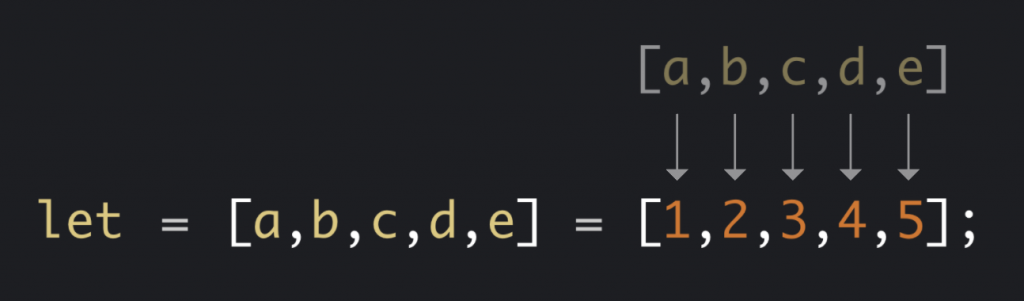#DAY 11
6
Modern Web

## Day11【ES6 小筆記】陣列的解構賦值-以哥哥的前女友為例（Array Destructuring）#### 陣列解構特性#### 基礎範例

``````let exGirl = ['蘋蘋','欣欣','雯雯','綺綺'];
``````

``````let one = exGirl;
let two = exGirl;
let three = exGirl;
let fore = exGirl;
``````

``````let [one, two, three, fore] = exGirl;
``````

``````let exGirl = ['蘋蘋','欣欣','雯雯','綺綺'];
let [one, , three, fore] = exGirl;
// one='蘋蘋'
// three='雯雯'
// fore='綺綺'
``````

#### 剩餘參數&陣列解構的運用

``````let exGirl = ['蘋蘋','欣欣','雯雯','綺綺'];
let [one, two , ...other] = exGirl;
console.log(other) // other = ['雯雯','綺綺']
``````

#### 預設值

``````let exGirl = ['蘋蘋','欣欣','雯雯','綺綺'];
let [one, two , three , fore , five ="月月"] = exGirl;
console.log(five) // five = 月月
``````

#### 變數交換

``````var one = '蘋蘋';
var two = '欣欣';

[one, two] = [two, one];
console.log(one); // one = 欣欣
console.log(two); // two = 蘋蘋
``````

#### 字串拆解

``````let please = '格幫我買飲料';
[a, b, c, d, e,f] = please;
// a=格 b=幫 c=我 d=買 e=飲 f=料
``````

MDN
CodecastsJS

ESLint airbnb 撰寫規則
5.2 使用陣列解構子### 7 則留言

1

0

0

0

0

0

XDDD 我有！！！本篇是真實故事

0